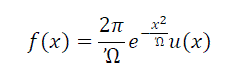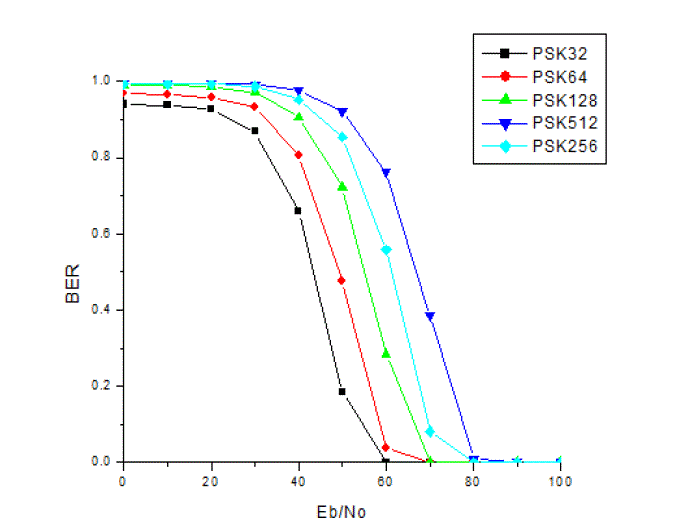ISSN ONLINE(2320-9801) PRINT (2320-9798)

All submissions of the EM system will be redirected to Online Manuscript Submission System. Authors are requested to submit articles directly to Online Manuscript Submission System of respective journal.

# Performance of M-PSK Schemes under Rayleigh Fading Channels

 Vivekanand, KangkanThakuria, AbhijyotiGhosh Student, Dept. of E.C.E., Mizoram University, Tanhril, Mizoram, India Student, Dept. of E.C.E., Mizoram University, Tanhril, Mizoram, India Assistant Professor, Dept. of E.C.E., Mizoram University, Tanhril, Mizoram, India Related article at Pubmed, Scholar Google

Visit for more related articles at International Journal of Innovative Research in Computer and Communication Engineering

## Abstract

With the evaluation of new mobile communication technologies radio spectrum is becoming valuable for service providers. So there is always a search for digital modulation technique that is bandwidth efficient and has low bit error rate at a relatively low signal to noise power. Taking this requirement in our mind, in this paper we provide a general theoretical analysis of various M-ary PSK ( M = 32.64,128,256 & 512 ) modulation schemes using MATLAB Simulink model taking BER as measure of performance when the system is subjected to multipath Rayleigh fading channel. Result presented in this paper proves that increasing the value of M results in increase of BER with the increase in data rate.

### Keywords

M-PSK, BER, AWGN channel, Fading channel, data rate

### INTRODUCTION

Modern wireless Communication system use digital modulation schemes because of its many advantages over the analog modulation schemes. The choice of modulation technique varies with applications according to its requirement. Digital modulation technique has different performance characteristics i.e. power efficiency, Bandwidth efficiency, Error rate, cost etc which are contradictory to each other. At the same time we cannot optimize each character. The choice of modulation is based on finding the technique that achieves the best trade-off between these requirements the different modulation techniques are Amplitude Shift Keying (ASK), Frequency Shift Keying (FSK), Phase Shift Keying (PSK) and Quadrature Amplitude Modulation (QAM). In digital modulation message signal is digital in nature and the carrier is analog in nature. The parameters of analog carrier signal like amplitude, frequency and phase are varying according to the base band digital signal. The amplitude modulated analog carriers in case of ASK are poor in quality and therefore used in low speed communication process like telemetry circuits. The error performance of FSK in presence of channel noise is very poor. Among the different digital modulation techniques Phase Shift Keying (PSK) has better error performance and band width efficiency. In PSK the phase of the analog carrier is varied according to the baseband digital signal to represent two or more different signal elements keeping the peak amplitude and fundamental frequency of the carrier fixed .

### RELATED WORK

M-ary modulation schemes are analyzed because of its various useful performance characteristics. In  it has been reported that the performance of M-ary modulations schemes like MPSK, MQAM, and MFSK for transmitting audio signal over Additive Gaussian Noise (AWGN) channel is better than low level modulation techniques in terms of bit error probability as a function of SNR. The error performance of 4-PAM, 8-PSK and 16-QAM over AWGN channel has been studied in  and it has shown that error performance is improving as the value M increases. In  comparative performance analysis of basic M-ary PSK modulation schemes like BPSK, QPSK and 8-PSK in AWGN channel has been done in  and it has shown that error rate increases with the increase value of M. A study of Bit Error Rate (BER) between BPSK, QPSK and GMSK over Rayleigh Fading Channel has been reported in  and using
simulation results it is shown that BER of GMSK is lower than BPSK and QPSK. QPSK is prone to bit error in fading channel than BPSK . But most of the articles investigate the error performance in AWGN channel with low level M ary modulation schemes.
Practically now a days there is a huge demand of such communication system which can provide higher data rate with mobility. Higher data rate means more numbers of bit should be send per second, which can be obtain by increasing the value of M in M-ary modulation schemes and to provide the mobility wireless communication is the best option. Wireless communication means wireless channel and wireless channel can be studied by multipath fading channel. For these purpose a study of error performance of higher order M-ary PSK ( M= 32, 54, 128, 256 and 512) modulation schemes over multipath Rayleigh fading channel has been presented in this article.

### M-ARY PSK

M-ary signalling is the higher version of basic digital modulation techniques where two or more bits of message signal are grouped together to make symbol and one of the M possible signals are transmitted during one symbol duration (Ts). The number of possible signals is M=2N where N is the number of bits in one symbol .
In M-ary PSK, the carrier phase takes on one of M possible values, namelyThe modulated waveform can be expressed asWhere Es= (log2 M)Ebis the energy per symbol and Ts=(log2 M)Tb is the symbol period . Power spectral density of M-ary PSK isThe above equation gives the power spectral density of baseband M-ary PSK ‘Tb’ is the duration of one bit. Fig1 shows the plot of SB(f) for M-ary PSK.
The spectrum of Fig1 is placed at the carrier frequency after modulation. Therefore the bandwidth required by the system is equal to the width of the main lobe i.e. 2fb/N Bandwidth requirement reduces as the number of successive bits (N) per symbol are increased, the bandwidth requirements reduces . Bit error rate of M-PSK in AWGN & fading channel is given byIn wireless communication, the emitted electromagnetic waves from transmitting antenna often do not reach the receiving antenna directly due to obstacles blocking the line-of-sight path. In fact, the received waves are a superposition of waves coming from all directions due to reflection, diffraction, and scattering caused by buildings, trees, and other obstacles. This effect is known as multipath propagation. The signal received at the receiver end after propagating through different path is basically attenuated, delayed, phase shifted version of the original signal. Such channels are called multipath fading channels. If the signal after passing through the fading channel is received via a line of sight path then the channel is called Ricean fading channel and if the signal is received via non line of sight path then the channel is called Rayleigh fading channel [7, 8].
If the transmitter and receiver are separated by large distance of few kilometres then propagation of the signal is described by large scale propagation model and fading is called large scale or macroscopic fading. Few examples of large scale fading are of satellite communication system and microwave radio links. When there is rapid variation of the signal over a short distance between the transmitter and the receiver; distance is of few wavelengths; such fading is called small scale fading or microscopic fading .
Microscopic fading comprises of rapid fluctuation of signal in space, time and frequency due to scattering objects in the channel. The scattered components when collected at the receiver end is described by the Rayleigh distributed function and is given bywhere Ω is average received power and u(x)is the unit step function.

### SYSTEM MODEL

The model  for the study of error performance of M-PSK (M = 32, 64, 128,256 and 512) is shown in Fig 2. In the system model the random integer generator generates a stream of random bits as 0 or 1. These bits are modulated using different M-PSK techniques in modulator block. The modulated signal passes through the channel characterized by multipath Rayleigh fading channel. The probability of bit error is computed by dividing the no. of errors to the total no. of bits sent. In this paper Bit Error Rate (BER) is considered as the measure of performance for M-ary PSK schemes (for M=32, 64,128, 256 &512) in Rayleigh fading channel.

### RESULT & DISCUSSION

The Bit Error Rate (BER) performance of M-PSK modulation techniques with respect to the signal to noise power (Eb/N0) ratio when the channel is characterized by multipath fading has been shown in Fig 3. From the Fig 3 it can be seen that as signal power is increased bit error rate (BER) decreases. The signal power is increased by increasing the value of Eb i.e. bit energy. The same characteristics i.e. decrease in error rate with increase in signal power has been seen with all the M-PSK techniques. From the same fig it can also seen that as the value of M in different M-PSK techniques increases, the error rate is also increases i.e. at Eb/N0 = 0dB, the error rate in 64-PSK (shown in red colour) is higher that the error rate in 32-PSK (shown in black colour). This is true for all other M-PSK techniques.
In  the error performance of different M-PSK in normal AWGN has been described. Comparing the error performance of M-PSK techniques in AWGN channel  & Rayleigh fading channel, for a constant value of M, BER in Rayleigh Fading Channel is much higher compare to BER in AWGN Channel. In case of both AWGN and multipath fading channel, it can be seen that for 32-PSK, error rate is maximum at Eb/No = 0dB. In AWGN channel  error rate is much lesser than error rate in Rayleigh fading channel. If we compare error performance presented for all other M-PSK techniques in AWGN channel  with the error performance in fading channel we can see that error rate in fading channel is higher than the error rate in AWGN channel.

### CONCLUSION

M-ary modulation techniques provide better bandwidth efficiency and higher data rate than normal modulation techniques. In this paper error performance of different M-ary PSK modulation techniques in Rayleigh fading channel has been studied. Here the value of M is chosen as 32, 64, 128, 256 and 512. In 32-PSK, 64-PSK, 128-PSK, 256-PSK and 512-PSK 5 bits, 6bits, 7bits, 8bits and 9 bits of original signal are combined to make the symbol respectively. As more numbers of bits are combined together to make the symbol data rate is also increases with the increase value of M. Bandwidth efficiency is also increasing with the increasing value of data rate. While data rate and bandwidth efficiency is increasing, which is advantage for any communication system at the same time the error performance is also increasing, which is disadvantages for any communication system. The error rate is decreasing with the increase in signal power.
The error rate is also depends on type of the channel. Multipath fading channel provides higher error rate on the transmitted signal than normal AWGN channel. Wireless channels are normally studied by different fading channels. Higher order modulation is preferred over short distance communication and lower order modulation techniques are preferred over long distance communication. While choosing a particular modulation technique for reliable communication through various channels, there should be always a trade-off between higher data rate and bit error rate.

### Figures at a glanceFigure 1 Figure 2 Figure 3